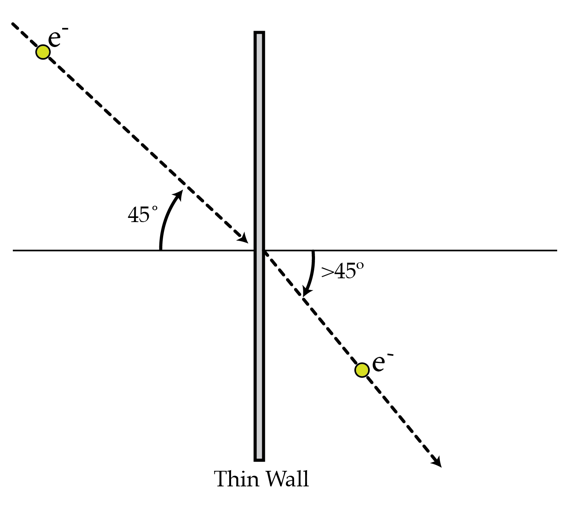# Electron's refraction

An electron with kinetic energy $K=5~\textrm{eV}$ moves in a region with constant potential $V_{1}=3~\textrm{V}$. The electron strikes a flat thin wall at $\alpha=45^{\circ}$ (measured with respect to the normal) and emerges in a different region with constant potential $V_{2}=2~\textrm{V}$. As a result, the electron refracts. Find the direction $\beta$ in degrees of the electron's velocity (measured with respect to the normal) in the second region. Assume that the total energy of the electron is conserved as it passes though the wall.

Hint: You may model the thin wall as a parallel plate capacitor.Details and assumptions

$1~\textrm{eV}= 1.6 \times 10^{-19}~\textrm{C} \cdot\textrm{ V}$.

×